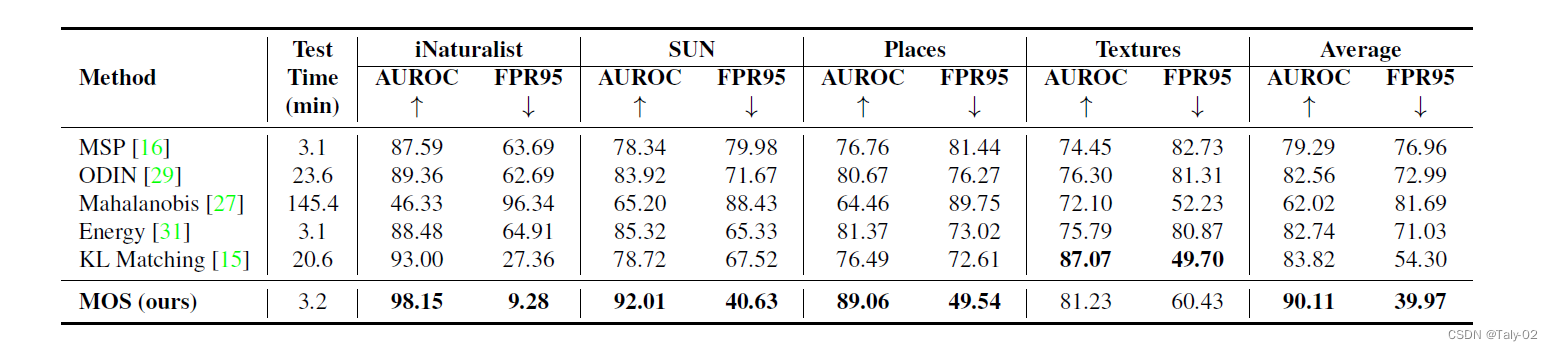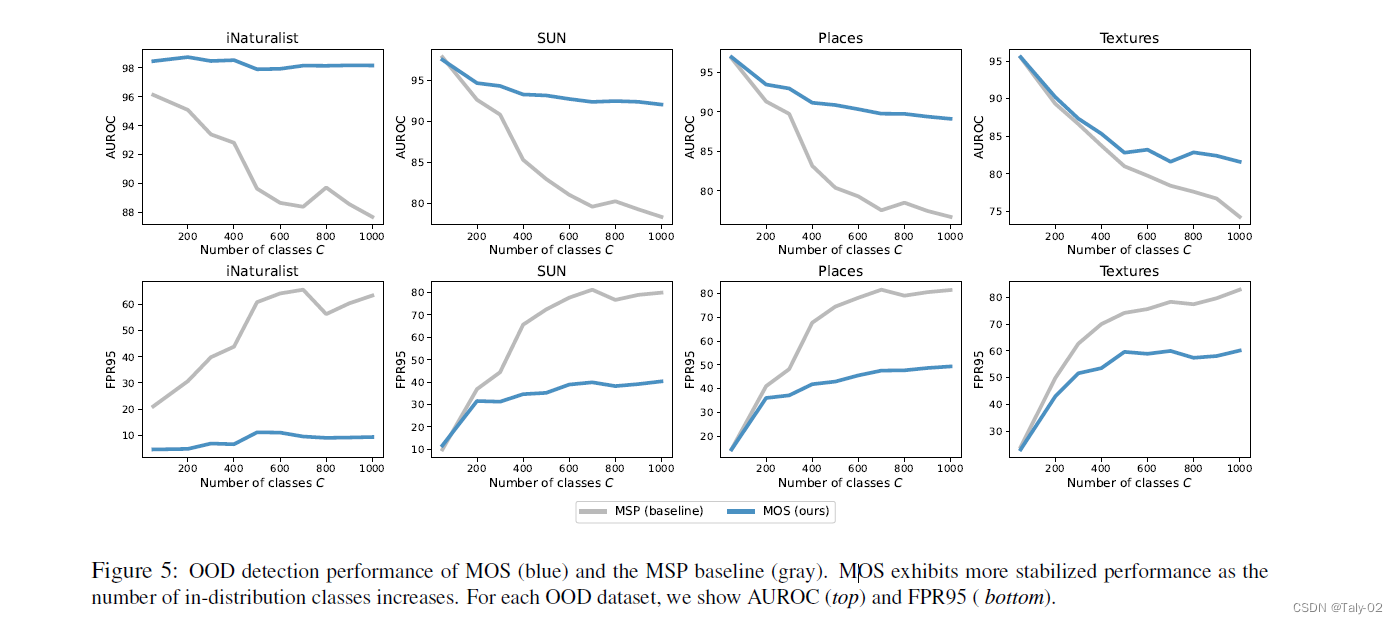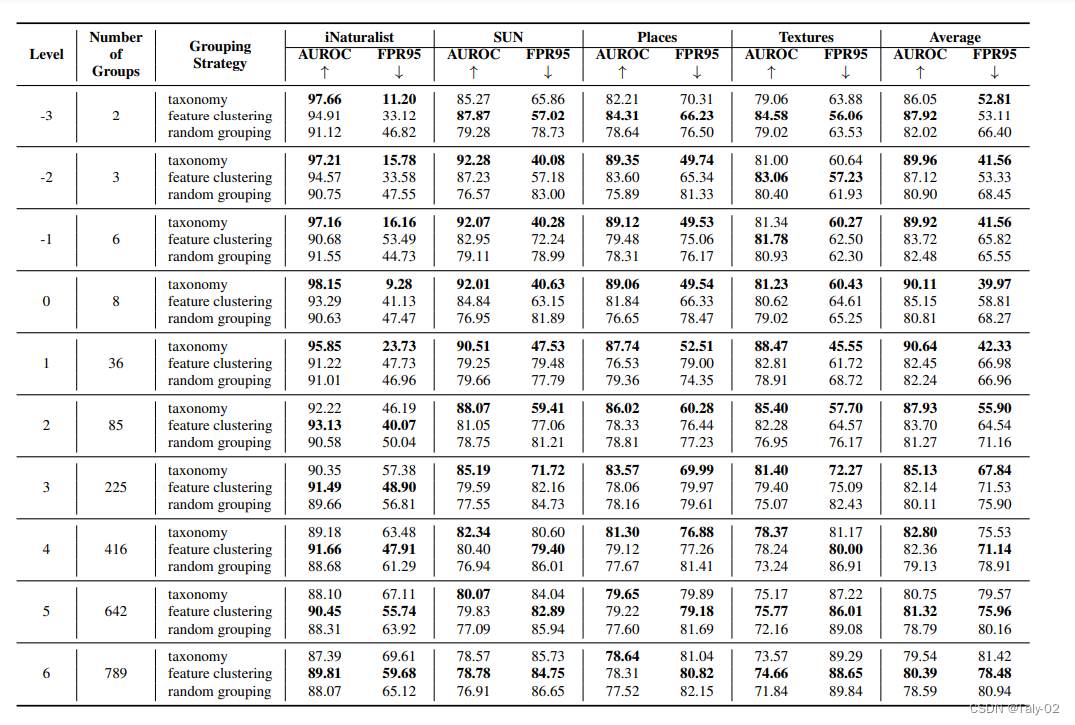# MOS: Towards Scaling Out-of-distribution Detection for Large Semantic Space CVPR 2022¶

### 2. 介绍¶

out -distribution (OOD)检测已经成为在开放世界中安全部署机器学习模型的一个核心挑战，在开放世界中，测试数据可能与训练数据分布不同。由于数据集中不可能枚举出所有真实世界可能出现的样本类别，所以在训练模型过程中，需要完成对于OOD样本的检测。然而，现有的解决方案主要是由小型、低分辨率的数据集驱动的，如CIFAR和MNIST。像自动驾驶汽车这样的部署系统通常对分辨率更高的图像进行操作，感知的环境类别也更多。因此，针对大规模图像分类任务的OOD检测算法的开发和评估存在一个关键的gap。本文首先志在提出一个benchmark实现大规模数据集上的OOD检测。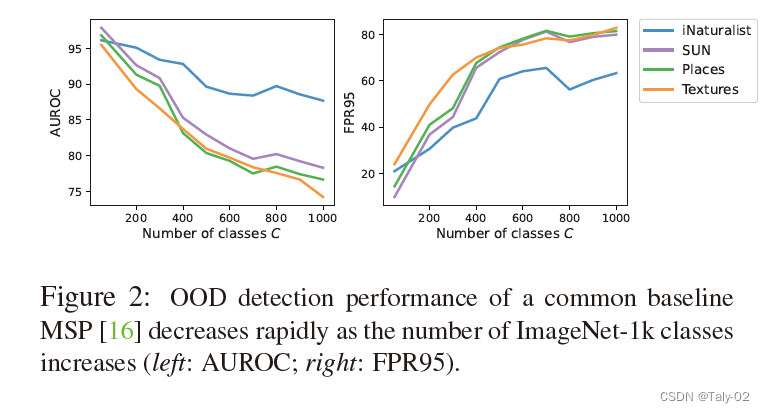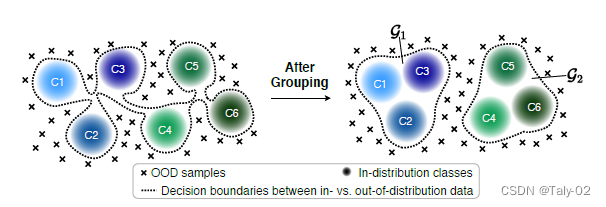### 3. 方法¶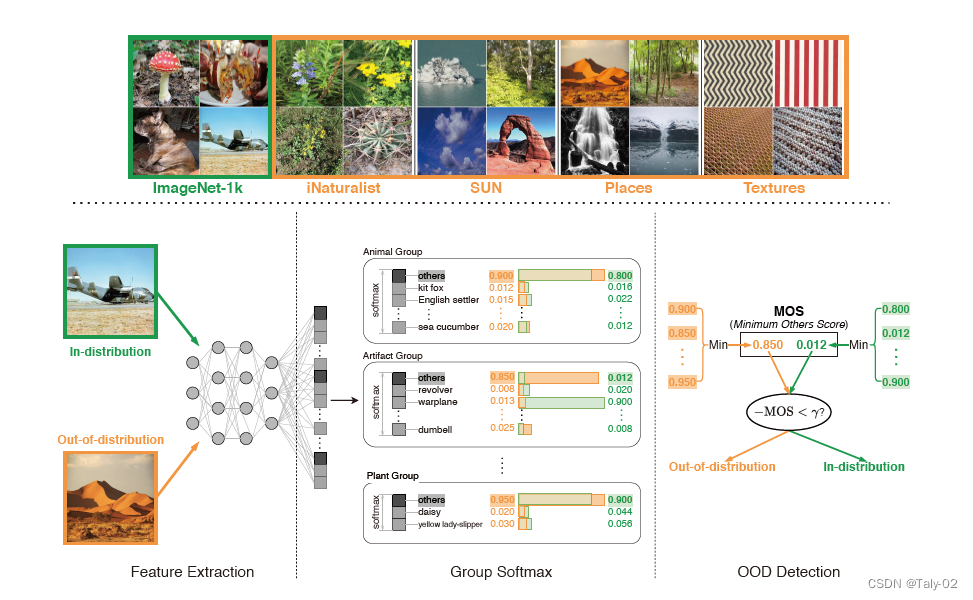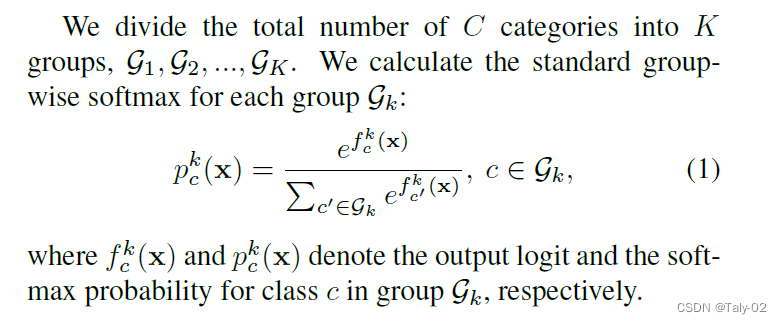\mathcal{L}_{G S}=-\frac{1}{N} \sum_{n=1}^{N} \sum_{k=1}^{K} \sum_{c \in \mathcal{G}_{k}} y_{c}^{k} \log \left(p_{c}^{k}(\mathbf{x})\right),

\hat{p}^{k}=\max _{c \in \mathcal{G}_{k}^{\prime}} p_{c}^{k}(\mathrm{x}), \hat{c}^{k}=\underset{c \in \mathcal{G}_{k}^{\prime}}{\arg \max } p_{c}^{k}(\mathrm{x}) .

k_{*}=\underset{1 \leq k \leq K}{\arg \max } \hat{p}^{k} .

how do different grouping strategies affect the performance of OOD detection?

• 根据标签已知的信息：比如大家都知道ImageNet-1k就是根据WordNet中的信息进行层次化标注的，所以其实不同类别是有相应的父类的，所以可以直接使用相应的标注作为grouping的根据。
• 根据特征聚类：自然是根据训练模型的特征，距离比较近的归为一个group，比较远的归为不同的group。
• 随机分组：这个自然是最简单的对照组。

### 4. 实验¶